﻿ 同步挤压改进短时傅里叶变换分频相干技术在断裂识别中的应用
 石油地球物理勘探2019, Vol. 54Issue (4): 860-866  DOI: 10.13810/j.cnki.issn.1000-7210.2019.04.0170文章快速检索 高级检索

### 引用本文YAN Haitao, ZHOU Huai-lai, Niu Cong, Wu Nanke, ZHOU Jian. Fault identification with short-time Fourier transform frequency-decomposed coherence improved by synchronous extrusion. Oil Geophysical Prospecting, 2019, 54(4): 860-866. DOI: 10.13810/j.cnki.issn.1000-7210.2019.04.017.### 作者简介严海滔, 四川省成都市二仙桥东三路1号成都理工大学地球物理学院, 610059。Email:YanHaiTaocdut@163.com

### 文章历史

① 成都理工大学地球物理学院, 四川成都 610059;
② 油气藏地质及开发工程国家重点实验室, 四川成都 610059;
③ 中海油研究总院, 北京 100028

Fault identification with short-time Fourier transform frequency-decomposed coherence improved by synchronous extrusion
YAN Haitao, ZHOU Huai-lai①② , Niu Cong , Wu Nanke , ZHOU Jian
① College of Geophysics, Chengdu University of Technology, Chengdu, Shichuan 610059, China;
② State Key Laboratory of Oil and Gas Reservoir Geology and Exploitation, Chengdu University of Technology, Chengdu, Shichuan 610059, China;
③ CNOOC Research Institute, Beijing 100028, China
Abstract: Limited by low resolution seismic data and background noise caused by stratigraphic occurrence, minor faults and fractures are very difficult to be accurately identified.To solve this issue, an approach is proposed in the paper.The high-frequency multi-resolution of short-time Fourier transform improved by synchronous extrusion decomposes seismic data volume spectrum into single-frequency data of different frequencies, and the multi-trace tilt-oriented coherence is used to cohe-rently calculate the single-frequency data volume.The proposed approach can effectively eliminate the background noise and improve seismic data resolution.The multi-trace tilt-oriented coherence can accurately characterize minor faults and fractures.This proposed approach is successfully applied to the fault identification in some work areas in the South China Sea and rather good results are achieved.
Keywords: fault identification    simultaneous-extrusion improved short-time Fourier transform    frequency-decomposed    coherence
0 引言

1999年Partyka等提出了谱分解方法，将全频带的地震数据体分解为不同频率的数据体，以突出地震数据中一些不同尺度的地质体。2009年Zeng等发现某些单频地震数据体比常规的全频带数据体对地质体的边界、范围的刻画更加清晰，细节更加丰富，为频率域的断裂解释提供了新的思路。但是受时频分析算法分辨率的影响，结果往往出现一些误差，因此，只有利用高时频分辨率算法在时频域的断裂刻画，才能得到更为准确的结果。

2011年Daubechies等在小波变换的基础上提出了同步挤压变换方法，在时频域内对小波变换后的时频谱值挤压和重排，信号的瞬时频率更加接近真实频率，提高了信号时频分析的时频聚焦能力。2017年Yu等拓展短时傅里叶变换(STFT)窗函数，提出了改进短时傅里叶变换，分辨率更为集中。但是，它仍受到海森堡不确定性原理的制约以及原因不明的交叉项影响。据此，在同步挤压算法的启发下，将改进短时傅里叶变换(GSTFT)与同步挤压变换算法进行结合，发展了同步挤压改进短时傅里叶变换(SSTFT)。

1 技术与原理 1.1 短时傅里叶变换

 ${\text{STFT}}\left( {t, f} \right) = \int_{-\infty }^\infty {x\left( t \right)g\left( {t-\tau } \right)\exp \left( {-{\text{i}}2\pi ft} \right){\text{d}}t}$ (1)

 ${\text{STF}}{{\text{T}}_x}\left( {t, f} \right) = \sum\limits_{t =-\infty }^\infty {x\left( t \right)g\left( {t-\tau } \right){{\text{e}}^{-{\text{i}}ft}}}$ (2)
1.2 改进短时傅里叶变换

 $\begin{array}{l} {\rm{STFT}}\left( {t, f} \right) = \int_{- \infty }^\infty {x\left( t \right){{\left[{g\left( {t-\tau } \right){{\rm{e}}^{-{\rm{i}}ft}}} \right]}^*}{\rm{d}}t} \\ \;\;\;\;\;\;\;\;\;\;\;\;\;\;\;\;\; = \int_{ - \infty }^\infty {x\left( t \right){{\left[{{g_\omega }\left( t \right)} \right]}^*}{\rm{d}}t} \\ \;\;\;\;\;\;\;\;\;\;\;\;\;\;\;\;\; = \frac{1}{{2\pi }}\int_{ - \infty }^\infty {\hat x\left( \xi \right){{\left[{{{\hat g}_\omega }\left( \xi \right)} \right]}^*}{\rm{d}}\xi } \end{array}$ (3)

 ${\hat g_\omega }\left( \xi \right) = \int_{-\infty }^\infty {g\left( {t-r} \right){{\rm{e}}^{{\rm{i}}ft}}{{\rm{e}}^{-{\rm{i}}\xi t}}{\rm{d}}t}$ (4)

t-r=r′，则

 $\begin{array}{l} {{\hat g}_w}\left( \xi \right) = \int_{-\infty }^\infty {g\left( {r'} \right){{\rm{e}}^{{\rm{i}}f\left( {r + r'} \right)}}{{\rm{e}}^{-{\rm{i}}\xi \left( {r + r'} \right)}}{\rm{d}}r'} \\ \;\;\;\;\;\;\;\;\;\; = {{\rm{e}}^{{\rm{i}}fr-{\rm{i}}\xi r}}\int_{ - \infty }^\infty {g\left( {r'} \right){{\rm{e}}^{{\rm{i}}fr' - {\rm{i}}\xi r'}}{\rm{d}}r'} \\ \;\;\;\;\;\;\;\;\;\; = {{\rm{e}}^{{\rm{i}}fr - {\rm{i}}\xi r}}\hat g\left( {f - \xi } \right) \end{array}$ (5)

 $\begin{array}{l} {\rm{STFT}}\left( {t, f} \right) = \frac{1}{{2\pi }}\int_{- \infty }^\infty {\hat x\left( \xi \right){{\left[{{{\rm{e}}^{{\rm{i}}ft-{\rm{i}}\xi t}}\hat g\left( {f-\xi } \right)} \right]}^*}{\rm{d}}\xi } \\ \;\;\;\;\;\;\;\;\;\;\;\;\;\;\; = {{\rm{e}}^{ -{\rm{i}}ft}}\frac{1}{{2\pi }}\int_{ -\infty }^\infty {\hat x\left( \xi \right)\hat g\left( {f -\xi } \right){{\rm{e}}^{{\rm{i}}f\xi t}}{\rm{d}}\xi } \end{array}$ (6)

 ${\rm{GSTFT}}\left( {t, f} \right) = \frac{1}{{2\pi }}\int_{-\infty }^\infty {\hat x\left( \xi \right)\hat g\left( {f-\xi } \right){{\rm{e}}^{{\rm{i}}\xi t}}{\rm{d}}\xi }$ (7)
1.3 同步挤压改进短时傅里叶变换

 $\begin{array}{l} {\partial _t}{\rm{GSTFT}}\left( {t, f} \right) = {\partial _t}\left[{A\hat g\left( {f-{f_0}} \right){{\rm{e}}^{{\rm{i}}{f_0}t}}} \right]\\ \;\;\;\;\;\;\;\;\;\;\;\;\;\;\;\;\;\;\;\;\;\; = {\rm{GSTFT}}\left( {t, f} \right){\rm{i}}{f_0} \end{array}$ (8)

 ${f_0}\left( {t, f} \right) =-{\rm{i}}\frac{{{\partial _t}{\rm{GSTFT}}\left( {t, f} \right)}}{{{\rm{GSTFT}}\left( {t, f} \right)}}$ (9)

 ${\rm{SSTFT}}\left( {t, \eta } \right) = \int_{- \infty }^\infty {{\rm{GSTFT}}\left( {t, f} \right)\delta \left[{\eta-{f_0}\left( {t, f} \right)} \right]{\rm{d}}f}$ (10)

1.4 多道倾角导向相干技术

2 应用效果 2.1 时频效果分析

 $\begin{array}{l} x\left( t \right) = \cos \left[{2\pi \left( {10 + \frac{t}{{6.2}}} \right)\frac{t}{{256}}} \right] + \\ \;\;\;\;\;\;\;\;\;\cos \left[{2\pi \left( {91-\frac{t}{{6.2}}} \right)\frac{t}{{256}}} \right]\;\;\;\;\;\;\;\;\;\;\;0 \le t \le 256 \end{array}$ (11)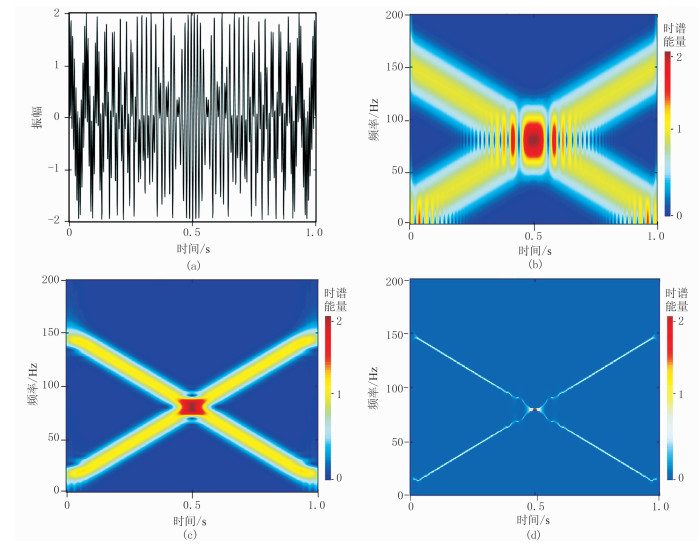图 1 合成信号及不同算法得到的时频谱 (a)原始合成信号；(b)STFT；(c)GSTFT；(d)SSTFT
2.2 抗噪性研究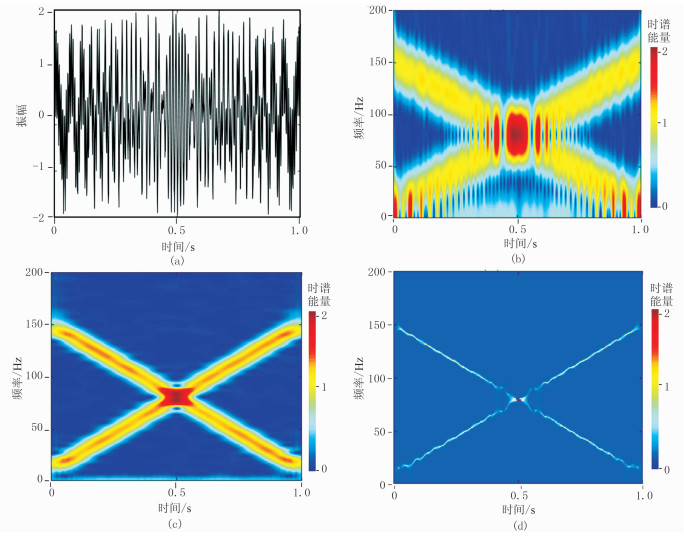图 2 加噪信号及不同算法得到的时频谱 (a)原始加噪信号；(b)STFT；(c)GSTFT；(d)SSTFT

2.3 多道倾角导向相干技术应用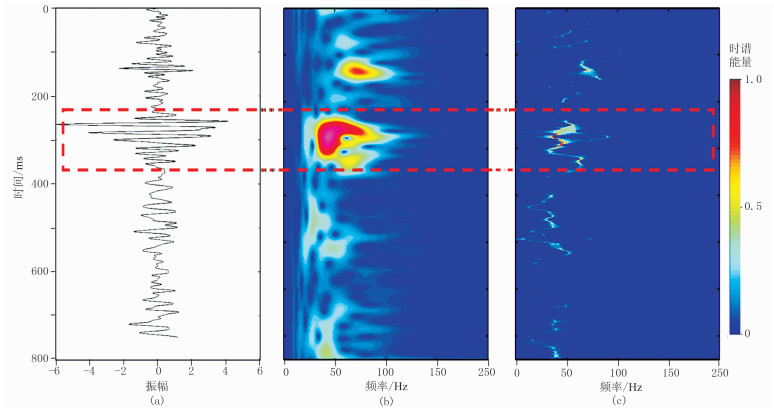图 3 井旁地震道及不同算法得到的时频谱 (a)井旁地震道；(b)STFT；(c)SSTFT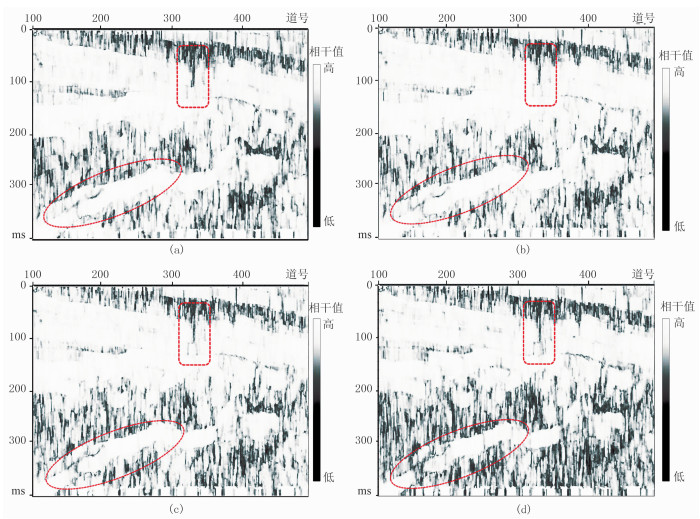图 4 单频相干属性剖面 (a)25Hz；(b)30Hz；(c)40Hz；(d)60Hz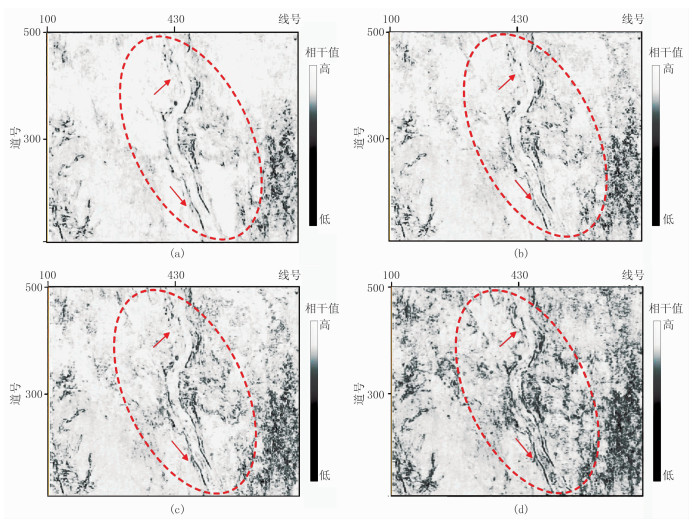图 5 单频相干属性沿层切片 (a)25Hz；(b)30Hz；(c)40Hz；(d)60Hz
3 结束语

  Bahorich M, Farmer S.3D seismic discontinuity for faults and stratigraphic features: the coherence cube[C]. SEG Technical Program Expanded Abstracts, 1995, 14: 1053-1058.  Marfurt K J, Kirlin R L, Famrer S L, et al. 3-D seismic attributes using a semblance-based coherency algoritmh[J]. Geophysics, 1998, 63(4): 1150-1165. DOI:10.1190/1.1444415  Gersztenkorn A, Marfurt K J. Eigenstructure-based coherence computation as an aid to 3-D structural and srtatigraphic mapping[J]. Geophysics, 1999, 64(5): 1468-1479. DOI:10.1190/1.1444651  王西文, 杨孔庆, 刘全新, 等. 基于小波变换的地震相干体算法的应用[J]. 石油地球物理勘探, 2002, 37(4): 328-331. WANG Xiwen, YANG Kongqing, LIU Quanxin, et al. Research on algorithm of seismic coherent cube based on wavelet transftorm[J]. Oil Geophysical Prospecting, 2002, 37(4): 328-331. DOI:10.3321/j.issn:1000-7210.2002.04.005  Partyka G, Gridley J, Lopez J. Interpretational applications of spectral decomposition in reservoir characterization[J]. The Leading Edge, 1999, 18(3): 353-360. DOI:10.1190/1.1438295  Zeng H, John A.Frequency-dependent seismic strati-graphy[C]. SEG Technical Program Expanded Abstracts, 2009, 28: 1097-1101.  Daubechies I, Lu J, Wu H T. Synchrosqueezed wavelet transforms: An empirical mode decomposition-like tool[J]. Applied and Computational Harmonic Analysis, 2011, 30(2): 243-261.  Yu G, Yu M, Xu C. Synchroextracting transform[J]. IEEE Transactions on Industrial Electronics, 2017, 64(10): 8042-8054. DOI:10.1109/TIE.2017.2696503  韩磊, 张宏, 王劲松, 等. 分频相干技术在复杂断裂解释中的应用[J]. 复杂油气藏, 2016, 9(4): 16-21. HAN Lei, ZHANG Hong, WANG Jinsong, et al. Discrete frequency coherency technology for interpreting complicated faults and its application[J]. Complex Hydrocarbon Reservoirs, 2016, 9(4): 16-21.  冯连勇, 黎莉, 裴勇. 胜利油田岩性油藏勘探中的地震技术[J]. 石油地球物理勘探, 2006, 41(2): 211-215. FENG Lianyong, LI Li, PEI Yong. Seismic technology in lithologic reservoir prospecting of Shengli oilfield[J]. Oil Geophysical Prospecting, 2006, 41(2): 211-215. DOI:10.3321/j.issn:1000-7210.2006.02.019  刘传虎. 地震相干分析技术在裂缝油气藏预测中的应用[J]. 石油地球物理勘探, 2001, 36(2): 238-244. LIU Chuanhu. Application of seismic coherent analysis technology to prediction of fractured reservoir[J]. Oil Geophysical Prospecting, 2001, 36(2): 238-244. DOI:10.3321/j.issn:1000-7210.2001.02.015  许海涛, 毕建军, 唐红光, 等. 数学形态学在地震相干属性提取中的应用[J]. 石油地球物理勘探, 2010, 45(4): 482-484. XU Haitao, BI Jianjun, TANG Hongguang, et al. Application of mathematical morphology in extraction of seismic coherent attributes[J]. Oil Geophysical Prospecting, 2010, 45(4): 482-484.  常旭, 刘伊克, 王辉, 等. 地震相干偏移与数据自参照偏移的关系[J]. 地球物理学报, 2009, 52(11): 2840-2845. CHANG Xu, LIU Yike, WANG Hui, et al. Seismic interferometric migration and data-referenced-only migration[J]. Chinese Journal of Geophysics, 2009, 52(11): 2840-2845.  王萍, 宋书君, 王新征, 等. 岩性油藏配套解释技术[J]. 石油地球物理勘探, 2003, 38(5): 557-560. WANG Ping, SONG Shujun, WANG Xinzheng, et al. Complete sets of interpretation technology for litho-logic reservoir[J]. Oil Geophysical Prospecting, 2003, 38(5): 557-560. DOI:10.3321/j.issn:1000-7210.2003.05.019  蔡涵鹏, 胡光岷, 贺振华, 等. 基于非线性变时窗相干算法的不连续性检测方法[J]. 石油地球物理勘探, 2016, 51(2): 371-375. CAI Hanpeng, HU Guangmin, HE Zhenhua, et al. Subtle discontinuity detection with nonlinear variable-time window coherency algorithm[J]. Oil Geophysical Prospecting, 2016, 51(2): 371-375.  问雪, 陈雪芳, 陈胜红, 等. 利用结构导向平滑方法解释断层[J]. 石油地球物理勘探, 2017, 52(1): 146-151. WEN Xue, CHEN Xuefang, CHEN Shenghong, et al. Fault interpretation based on structure-orientedsmoothing method[J]. Oil Geophysical Prospecting, 2017, 52(1): 146-151.  尹川, 杜向东, 赵汝敏, 等. 小波分频倾角相干在复杂断裂解释中的应用[J]. 石油地球物理勘探, 2015, 50(2): 346-350. YIN Chuan, DU Xiangdong, ZHAO Rumin, et al. Dip-steering similarity based on wavelet decomposition in complex fault interpretation[J]. Oil Geophysical Prospecting, 2015, 50(2): 346-350.  刘财, 邓馨卉, 郭智奇, 等. 基于岩石物理的页岩储层各向异性表征[J]. 石油地球物理勘探, 2018, 53(2): 339-346. LIU Cai, DENG Xinhui, GUO Zhiqi, et al. Shale reservoir anisotropic characterization based on rock physics[J]. Oil Geophysical Prospecting, 2018, 53(2): 339-346.  李军, 张军华, 龚明平, 等. 基于魔方矩阵的断层检测方法[J]. 石油地球物理勘探, 2018, 53(3): 552-557. LI Jun, ZHANG Junhua, GONG Mingping, et al. Fault detection based on magic matrix[J]. Oil Geophysical Prospecting, 2018, 53(3): 552-557.  王清振, 张金淼, 姜秀娣, 等. 利用梯度结构张量检测盐丘与断层[J]. 石油地球物理勘探, 2018, 53(4): 826-831. WANG Qingzhen, ZHANG Jinmiao, JIANG Xiudi, et al. Salt dome and fault detection based on the gradient-structure tensor[J]. Oil Geophysical Prospecting, 2018, 53(4): 826-831.